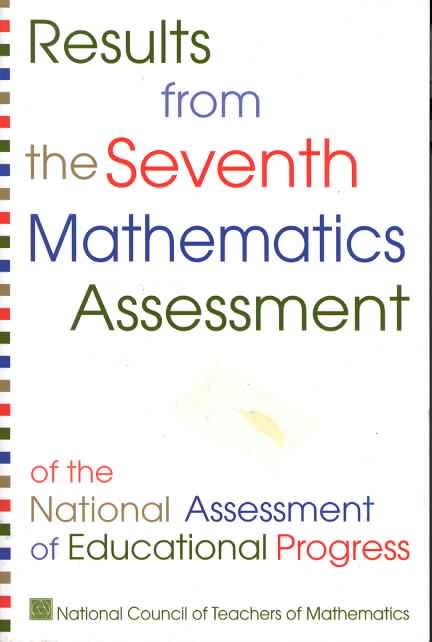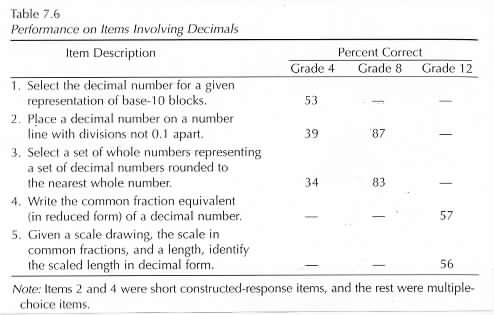Home
 SEVENTH MATHEMATICS ASSESSMENT .National Assessment of Educational Progress c. 2000 As with fractions an understanding of the symbolism for representing decimals is essential to developing understanding of symbolic operations with decimals. Students who do not understand decimal notation frequently resort to memorizing procedural rules (Bell Swan and Taylor 1981; Fischbein et al. 1985; Sackur-Grisvard and Leonard 1985; Resnick et al. 1989) rules that may be tied only to surface features of the tasks rather than to any underlying conceptual rationale (Heibert and Wearne 1985; Wearne and Hiebert What kind of understanding of decimal symbols did students display on the 1996 NAEP? Table 7.6 contains descriptions of the decimal items and the percents of students responding correctly. In item 1 fourth-grade students were asked to select the number associated with a base-10 block representation in which the 1 and the 0.01 blocks were identified. Approximately one-half of the fourth-grade students chose the correct response. About one-third of the students responded either by selecting the option with all of the digits to the right or to the left of the decimal point suggesting that they were probably using whole-number ideas to choose the number.Another way of assessing students’ understanding of decimal symbols is to ask them to write the number associated with a given place on a number line. For item 2 the divisions on the given number line were a multiple of 0.1 apart rather than 0.1. Almost 90 percent of the eighth-grade students but only about 40 percent of the fourth-grade students responded correctly to this item. This question is similar in some ways to the fraction items in which the region or number line was divided into a number of parts equal to a multiple of the denominator (see items 4 and 5 in table 7.1); similar in that the student has to attend to the number of divisions. The fourth-grade students found those fraction tasks to be difficult as well. A third way to assess decimal number knowledge is to ask students to round decimals to the nearest whole number as in item 3 in table 7.6. More of the fourth-grade students selected the response in which the decimal portion of the number was omitted (for example selecting 56 for the number 56.8) then responded correctly; 39 percent of the fourth-grade students made this error. However as in the previous example the eighth-grade students did not find this task difficult. A somewhat different way of assessing students’ understanding of symbols is to ask them to translate between different systems of notation. Of course students’ difficulties may be caused by a lack of understanding of either one or both of the systems so interpretations must be made with caution. There were two tasks which assessed knowledge of the relationship between decimals and common fractions both of which were administered only to twelfth-grade students (items 4 and 5). The first task item 4 asked the students to write the common fraction equivalent in reduced form of a given decimal. It would be of interest to know why so few of the twelfth-grade students responded correctly to this fairly straightforward question concerning the relationship between a decimal and a common fraction. It is possible that the students did not attend to the directions indicating they were to write the fraction in reduced form. The scoring of this short constructed-response item did not indicate what proportion of the students wrote an associated fraction that was not in reduced form. This item also was included on both the 1990 and 1992 assessments with approximately the same percent of students responding correctly. The other question assessing the relationship between fractions and decimals is item 5. This task was the more demanding of the two items in that the student had multiple steps to perform. In spite of this the twelfth-grade students were as successful on this item as they were on the rather straight-forward task of writing a fraction in reduced form that was equal to a given decimal. The item format may have played a role here; item 4 was a short constructed-response item and item 5 was multiple-choice.